Matplotlib: Lines, bars, pie charts and some other stuff

Why do I start from Matplotlib and data visualization? Well, maybe because my first academic degree is in Geodesy and Cartography, so visualized data tells me much more than just raw statistics, or maybe because this is just an easy starting point…

Anyway, It should be enough to just take a look at Anscombe’s Quartet (created by Francis Anscombe’a) or Datasaurus (created by Alberto Cairo) data sets, to clearly understand why statistical inference based just on tabular data might be misleading.

Now,  a few words on this post content. Visualization  this is a book-size topic, so first of all I had to pick one language and one library\module.  As python is my primary scripting language, there could be only one choice : “Matplotlib”.  Second thing is, that it won’t be a comprehensive post like ” from beginner to expert..” but rather a cheat sheet style post . If you want to know more about Matplotlib, then your best source of truth should be https://matplotlib.org/.

Alright, let’s get our hands dirty. For this post, I will use European Median equivalised net income (MENI) data set available on Eurostat home page.

Below code is just an extract from larger project code, which you can find here: https://github.com/kmakiel/Matplotlib_basics/blob/master/Matplotlib_basics.ipyn

1. Required python modules loading, turning off warning messages, switching to seaborn style, setting the backend of matplotlib to the ‘inline’ backend.

import pandas as pd
import numpy as np
import matplotlib.pyplot as plt
import seaborn as sns
import warnings
from scipy.interpolate import UnivariateSpline
from sklearn.metrics import r2_score

warnings.filterwarnings('ignore')
sns.set()
%matplotlib inline

After loading Median equivalised net income (MENI) data set to pandas dataframe and doing some small cleanup, we get a following data set structure ( below just few first rows..)

Age Indic_il Sex Unit Country 1995 1996 1997 1998 1999 2008 2009 2010 2011 2012 2013 2014 2015 2016 2017
0 TOTAL MED_E F EUR Austria 13394 14277 13841 13677 13888 18838 20030 20566 20949 21183 21629 22712 22741 23202 NaN
1 TOTAL MED_E F EUR Belgium 13085 13735 13707 13669 13885 17592 18851 18963 19380 19688 20878 21189 21012 21526 NaN
2 TOTAL MED_E F EUR Bulgaria NaN NaN NaN NaN NaN 2131 2762 2925 2814 2776 2860 3260 3236 3043 NaN
3 TOTAL MED_E F EUR Switzerland NaN NaN NaN NaN NaN 26114 28003 29780 32911 38156 39783 37278 38361 43091 NaN
4 TOTAL MED_E F EUR Cyprus NaN NaN NaN NaN NaN 15677 16005 15839 16580 16502 15437 14186 13618 13854 NaN

2. Scatter, line, bar and stack plots forming 2×2 grid (subplots )

Let’s start from a single observation (row) charts. As an example I will use MENI in Poland, given in polish zlotych, for all citizens regardless of their age or sex.

1995 1996 1997 1998 1999 2000 2001 2003 2004 2005 2008 2009 2010 2011 2012 2013 2014 2015 2016 2017
Country
Poland NaN NaN NaN NaN NaN NaN NaN NaN NaN 11467 15720 17903 19065 20075 20849 21610 22399 23247 24618 NaN
x = fplot.dropna(axis=1).columns.astype(int).tolist()         #explicitly storing x data set
y = (fplot.dropna(axis=1).values.astype(int)/12).tolist()  #explicitly storing y data set

fig, ax =plt.subplots(2, 2, figsize=(18,5), sharex='col', sharey='row')     #define subplots grid and figure size
fig.subplots_adjust(hspace=0.1, wspace=0.05)                                #set up space between subplots

plt.suptitle('Median equivalised monthly net income grow in Poland [PLN]', fontsize=16)
fig.text(0.5, 0.0, 'Year', ha='center', fontsize=14)
fig.text(0.07, 0.5, 'PLN', va='center', rotation='vertical', fontsize=14)

for i in range(2):                                                          #define ticks size for all axis
for j in range(2):
ax[i,j].tick_params(axis='both',which ='both', labelsize=13)

ax[0,0].plot(x,y,'o')                                                       #scatter plot
ax[1,0].plot(x,y,'-', color='green')                                        #line plot
ax[0,1].bar(x,y, color ='orange');                                          #bar plot
ax[1,1].stackplot(x,y, color ='red');                                       #stackplot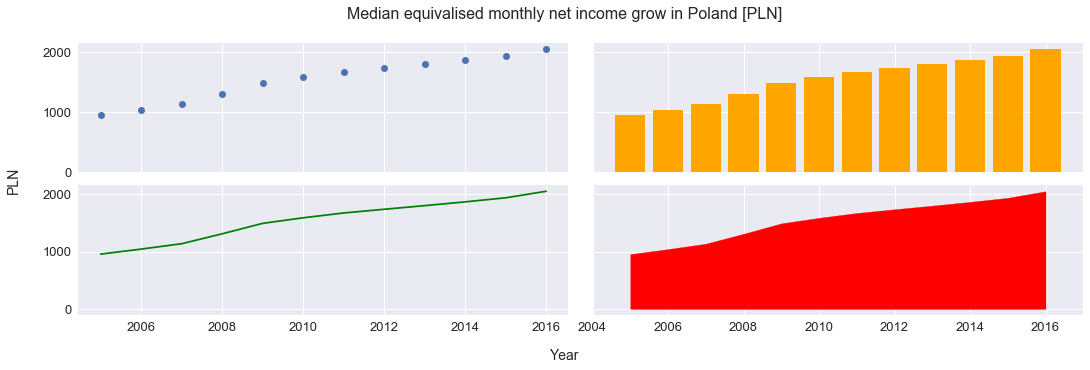3. Linear regression (polyfit), future MENI value prediction , coefficient of determination (r2_score).

#Fitting line into points
fit = np.polyfit(x, y, deg=1)
x_n=x + [2020,2021]
y_n =[i*fit +fit for i in x_n]

plt.figure(figsize=(18,5))
print('In 2020, median equivalised monthly net income in Poland will grow to ', str(round(y_n[-2],1)),\
'zlotych per month')

plt.title('Median equivalised monthly net income prediction with using linear regression', fontsize=16)
plt.xlabel('Year', fontsize=14)
plt.xticks(fontsize=13)
plt.ylabel("PLN", fontsize=14)
plt.yticks(fontsize=13)

plt.scatter(x,y, color = 'blue')
plt.scatter(x_n[-2],y_n[-2], color='red')
plt.plot(x_n,y_n, color='green');

coefficient_of_determination = r2_score(y, y_n[:-2])

print('Coefficient of determination [R2] is: ',round(coefficient_of_determination,2))
In 2020, median equivalised monthly net income in Poland will grow to  2495.6 zlotych per month
Coefficient of determination [R2] is:  0.97

Visually and looking at R2_score, linear regression on historical data seems to be a correct model (just a side note: in reality, as Poland is a rapidly developing market, which relatively recently woke up from communism,  so I think that long-term, MENI grow in Poland should speed up. Well, but I’m not a salary market analyst 😉 ). If we use linear regression for future values prediction, then in 2020, MENI should reach 2495.6 zl per month.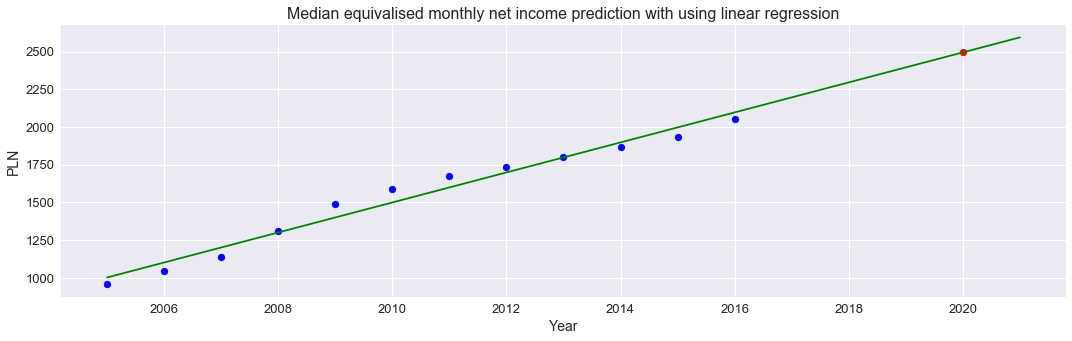4. Data comparison on bar and pie plots

We are already familiar with MENI in Poland, we have also predicted how it will look like in the near future, so let’s have a wider view now on the entire EU ( or actually on those countries, which are included in our data set). First let’s see how it looked like in 2016.
Country 2016
0 Switzerland 44253
1 Norway 39573
2 Luxembourg 33818
3 Denmark 28665
4 Sweden 25164
5 Austria 23694
6 Finland 23650
plt.figure(figsize=(18,5))
x = fplot.index.values.tolist()
y = fplot['2016 '].values.tolist()

plt.title('EU median equivalised annual net income comparison', fontsize=16)
plt.bar(x,y)
plt.xticks(x, fplot['Country'].values.tolist(), rotation='vertical', fontsize=14)
plt.ylabel('Euro',fontsize=14)
plt.yticks (fontsize=13);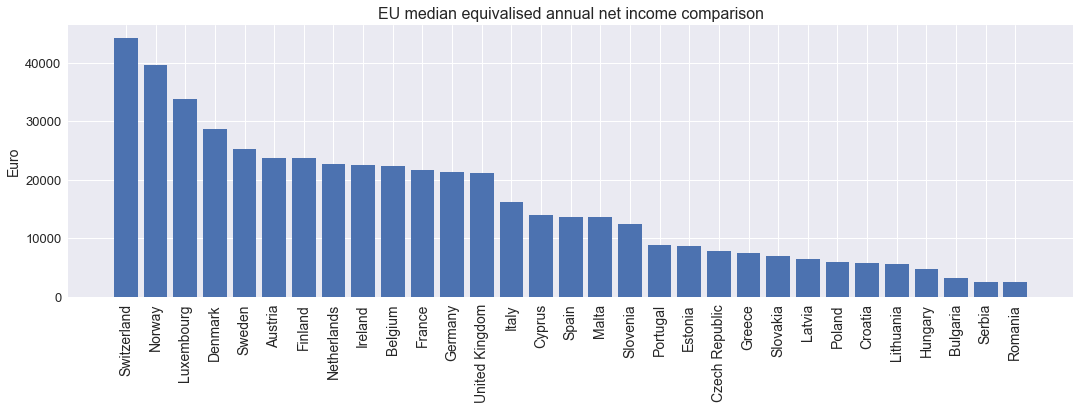plt.figure(figsize=(10,10))
# Create a palette with unique colors
colors=sns.set_palette(sns.color_palette("hls", len(fplot.index)))

labels =[str(i) + ": "+str(j) for i, j in zip(fplot['Country'].values.tolist(),y)]
plt.title('EU median equivalised annual net income comparison [Euro]', fontsize=16)
plt.pie(y, labels=labels,colors=colors, startangle=8);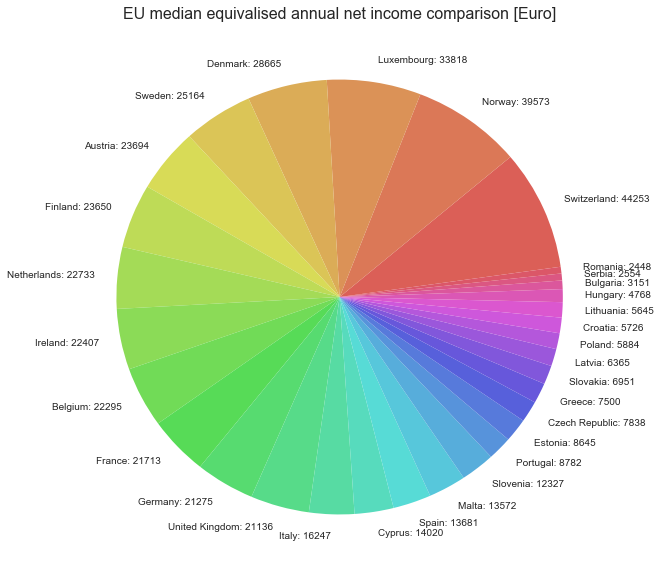I know, this pie chart is not very “fortunate” way for so much and this kind of data comparison, but I just did that for exercise (like everything on this playground … ).

5. Data comparison on histogram and boxplot.

If we don’t want to go into details, but we would just like to have some high level idea on how values are distributed, then we can do following:

fig, ax =plt.subplots(1, 2, figsize=(18,5))                               #define subplots grid and figure size
fig.subplots_adjust(wspace=0.2)                                           #set up space between subplots

plt.suptitle('EU median equivalised annual net income distribution', fontsize=16)

ax.hist(y, bins=(np.arange(0,50000, 5000)))                            #customize labels and ticks
ax.set_xlabel('Euro', fontsize=14)
ax.set_ylabel('Frequency', fontsize=14)
ax.tick_params(axis='both',which ='both', labelsize=13)
ax.set_xticks(np.arange(0,50000, 5000))
plt.setp( ax.xaxis.get_majorticklabels(), rotation=90)

ax.boxplot(y)
ax.set_xlabel('EU', fontsize=14)
ax.set_ylabel('Euro', fontsize=14)
ax.tick_params(axis='both',which ='both', labelsize=13);

for index, values in fplot[['2016 ']].describe()[3:].iterrows():          #display text with stats
ax.text(1.1,values, index +': '+ str(int(values)), fontsize=12)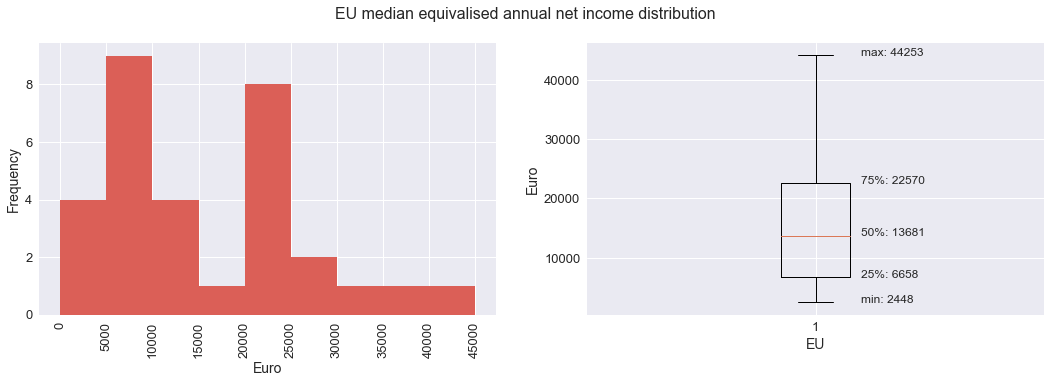From above charts we can see that the most common MENI threshold is 5000-10000 Euros annually and that in 75% of all* EU countries annual MENI is below 22570 Euro.

6. Time series, data gaps filling ( interpolating ), line smoothing (UnivariateSpline)

1995 1996 1997 1998 1999 2000 2001 2003 2004 2005 2008 2009 2010 2011 2012 2013 2014 2015 2016 2017
Country
Belgium 13405 14111 14089 14027 14200 14778 15492 15522 15674 16581 17985 19313 19464 20008 20280 21483 21705 21654 22295 NaN
Greece 5208 5467 5891 6413 6350 6924 7119 8206 8844 9417 10800 11496 11963 10985 9513 8371 7680 7520 7500 NaN
Spain 6173 6247 6619 6796 7485 8236 9034 NaN 10327 10453 13966 14795 14605 13929 13868 13524 13269 13352 13681 NaN
France 12653 13191 13353 13557 13814 14104 14889 NaN 15242 15946 18899 19644 19960 19995 20603 20924 21199 21415 21713 NaN
Poland NaN NaN NaN NaN NaN NaN NaN NaN NaN 2533 4155 5097 4405 5025 5060 5164 5336 5556 5884 NaN
Romania NaN NaN NaN NaN NaN NaN NaN NaN NaN NaN 1952 2172 2036 2091 2049 2016 2155 2315 2448 NaN
Slovakia NaN NaN NaN NaN NaN NaN NaN NaN NaN 2830 4792 5671 6117 6306 6927 6737 6809 6930 6951 NaN
United Kingdom 10429 10188 10962 13606 13814 15444 17724 NaN NaN 18540 18923 16262 17106 17136 19166 18694 20528 21028 21136 NaN

Let’s see how  MENI has changed over time in a few “randomly” selected countries.

plt.figure(figsize=(18,6))                                                     # Setting up plot size
sns.set_palette(sns.color_palette("hls", len(fplot.index)))                    # Create a palette with unique colors
for index, row in fplot.iterrows():                                            # Iterate through dataframe content
plt.plot(row, label =index)                                                # and plot data for each country

plt.title('Median equivalised annual net income grow comparison', fontsize=16)
plt.xlabel('Year', fontsize=14)
plt.ylabel('Euro', fontsize=14)
plt.tick_params(axis='both',which ='both', labelsize=13)
plt.legend(loc='lower left', bbox_to_anchor=(1, 0.5), ncol=1, prop={'size': 13});   # Plot Legend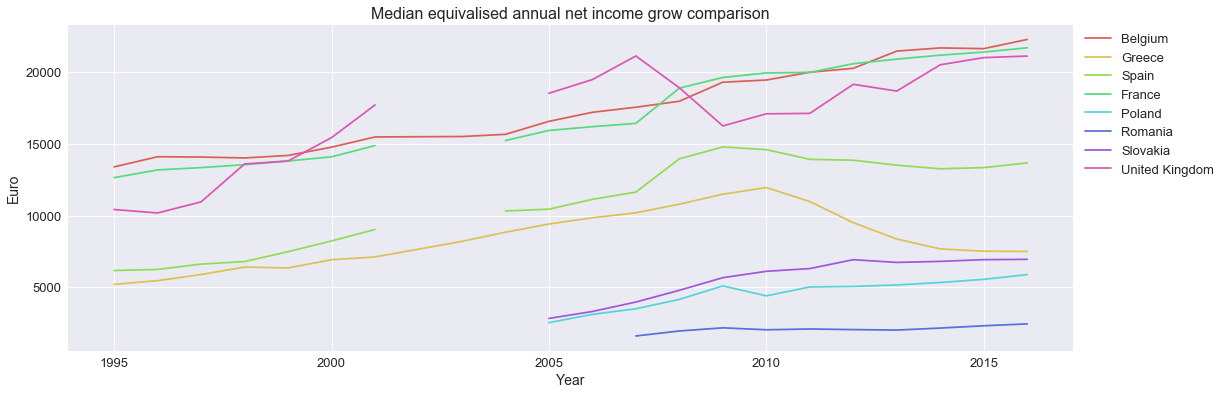Looks interesting (now we know why Greeks were complaining about forced savings introduced by government), and if this chart is just for analyzing purposes, without need for data visualization to wider audience, then I would leave it as it is. Even gap in data is some kind of data. With so little information, each smoothing step just leads to data precision degradation, but ok, let’s try to make this chart a little bit more “eye-catching”.
plt.figure(figsize=(18,6))                                                   # Setting up plot size

for index, row in fplot.iterrows():                                          # Interpolating NAN values
y=row.interpolate(method='linear').dropna().values
x=row.interpolate(method='linear').dropna().index.values.astype(float)

x_s = np.linspace(x.min(),x.max(),500)                                   # Smoothing lines with Spline
s = UnivariateSpline(x,y, k=3)
y_s = s(x_s)

plt.plot(x_s, y_s, label =index)

plt.title('Median equivalised annual net income grow comparison',{'size':'16'})
plt.xticks(np.arange(x.min(), x.max()+1, 2.0), rotation='vertical')        # Xticks rotation and distribution change
plt.xlabel('Year', fontsize=14)
plt.ylabel('Euro', fontsize=14)
plt.tick_params(axis='both',which ='both', labelsize=13)
plt.legend(loc='lower left', bbox_to_anchor=(1, 0.5), ncol=1, prop={'size': 13});  # Plot Legend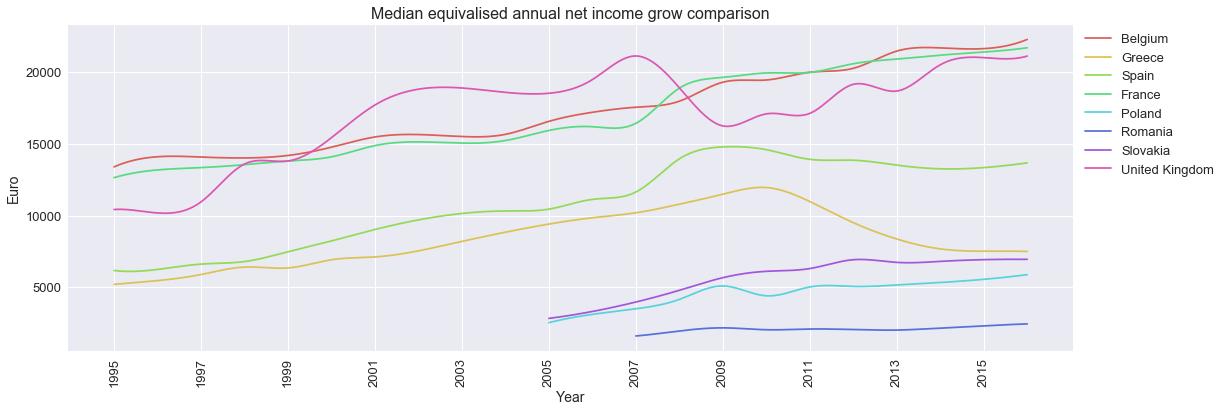And that’s it for a brief Matplotlib basic overview. Still with Excel we can produce the same charts or with some fancy tools like Tableau or Power BI, but if charts are just part of larger project, where code is written in python, then few lines of code and voilà.